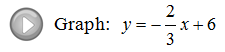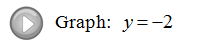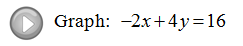## Algebra

Showing posts with label form. Show all posts
Showing posts with label form. Show all posts

### Graph using the y-intercept and Slope

Before we can understand our third method for graphing a line we must learn the concept of slope.  The slope is used to measure the steepness of a line. There are four cases.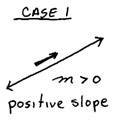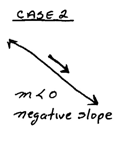Notice that the phrase "no slope" is not listed.  This phrase is ambiguous so do not use it. The slope is zero if the line is horizontal and the slope is undefined if the line is vertical.One way to determine the slope is to count the number of units that it takes to rise and run from point to point.  That is what you are asked to do if the graph is given.

* The vertical distance is the rise, up is positive and down is negative.
* The horizontal distance is the run, right is positive and left is negative.
* Pay close attention to the scale.When dealing with a negative fraction, remember that all of the following are equal:It is correct to use any one of these ratios for the slope.  You will get the same line.

If you are given two points,andthen you can calculate the slope algebraically using the slope formula,This is the difference in the y-values divided by the difference in the x-values.

Example: Find the slope of the line passing through (1,1) and (4,−1).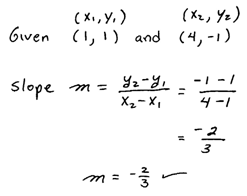It is best to write the formula down first and then substitute into it. Notice that we get the same answer as in the previous example.

Find the slope of given line.
Identify two points and then count to determine the rise and run.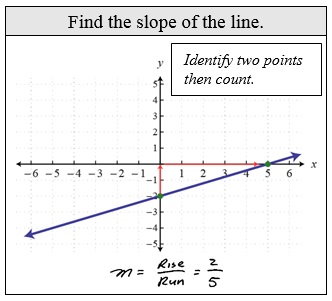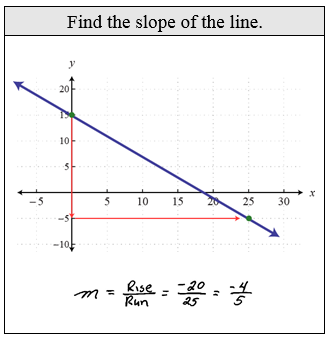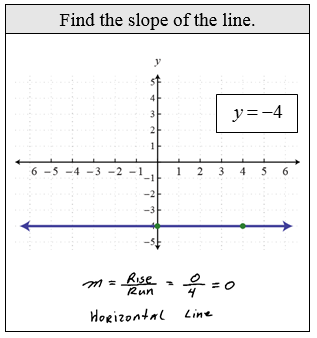Find the slope of the line passing through two given points.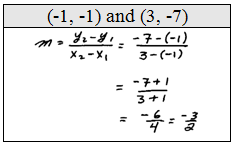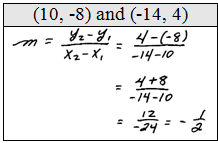You should always write in the formula before you plug into it. This makes your work more readable. Also, you might run into other books that refer to slope as change in y, or delta y, divided by the change in x, or delta x.

Word Problem: While driving on the Grapevine, Joe encountered a sign warning of a 6% downgrade in the road.  What does this say about the steepness of the road?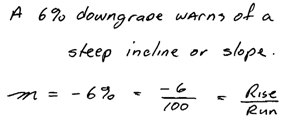Word Problem: The average tuition at a public four-year college was \$2,977 in 1995 and \$3,489 in 1998.  Find the rate at which tuition was increasing.

Since tuition depends on time, let x represent time in years and let y represent cost in dollars.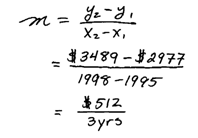Our final method for graphing lines will, in most cases, be the method of choice.  All lines are completely determined by their y-intercept and slope.  Once a linear equation is in slope-intercept form - graphing it becomes easy.Always start with the y-intercept and mark off the slope from there.  If you continue marking off the slope you can plot as many points as you wish.
Example: Graph y = −2/3 x + 4.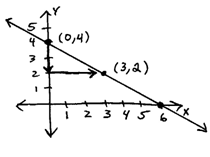Notice that if you continue marking off the slope in this particular example you will get the x-intercept, (6,0).  This is very special and does not always happen.

Graph the line.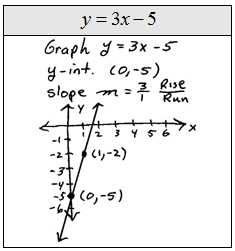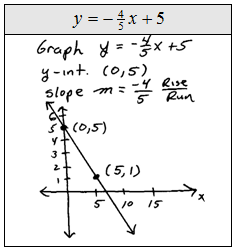Since two points determine a line it is nice to label at least two points on all of our graphs, even if it does not ask for us to do so.